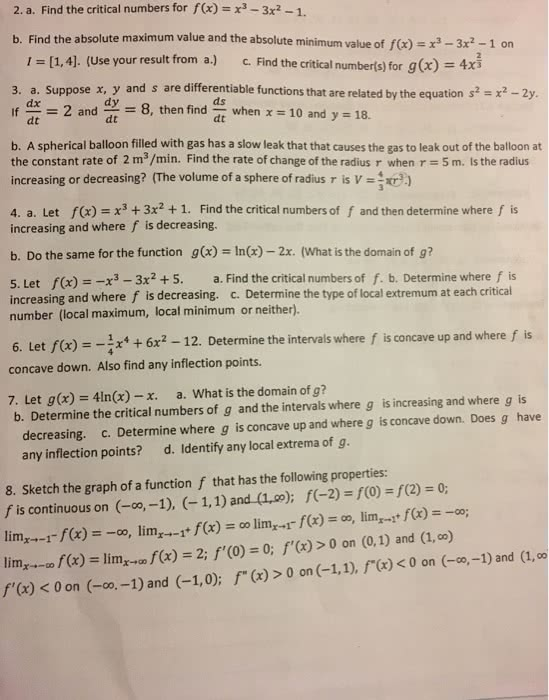1
0
watching
111
views13 Nov 2019

# Please answer numbers 2c, 3a, and 3b. Please and thank you.2. a. Find the critical numbers for fx)x3-3x2-1. your result from a.) 2 and 8, then finda when Find the absolute maximum value and the absolute minimum value of f(x) = x3-3x2-1 on 1 = [1,4]. (Use b. c. Find the critical number(s) for g (x) = 4x3 3. a. Suppose x, y and s are differentiable functions that are related by the equation s2 - x2 -2y dx ds 10 and y 18. dt dt b. A spherical balloon filled with gas has a slow leak that that causes the gas to leak out of the balloon at the constant rate of 2 m3/min. Find the rate of change of the radius r when r = 5 m. Is the radius increasing or decreasing? (The volume of a sphere of radius r is V = 4. a. Let fx)3+3x2 +1. Find the critical numbers of f and then determine where f is increasing and where f is decreasing. b. Do the same for the function gx) -InGx)-2x. (What is the domain of g? 5. Let f(x) =-x3-3x2 + 5. a. Find the critical numbers of f. b. Determine where f is increasing and where f is decreasing. c. Determine the type of local extremum at each critical number (local maximum, local minimum or neither). 6. Let f(x) =-4x4 + 6x2-12. Determine the intervals where f is concave up and where f is concave down. Also find any inflection points. 7. Let g(x) = 41n(x)-x. a. What is the dornain of g? b. Determine the critical numbers of g and the intervals where g is increasing and where g is decreasing. c. Determine where g is concave up and where g is concave down. Does g have any inflection points? d. Identify any local extrema of g. 8. Sketch the graph of a function f that has the following properties: f is continuous on (-oo,-1), (-1,1) and (1,co); f(-2)s ro)s f(2) = 0; lim,--cof(x) = limxâaf(x) = 2; f,(0) = 0; f,(x) >0 on (0,1) and (1.00) f(x) 0 on (-1,1), f-(x) Comments